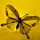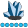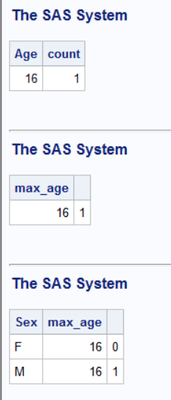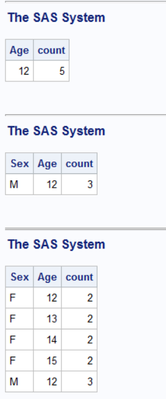## Count maximum age

``````proc sql;
select sex,age,count(*) as max_agecnt
from sashelp.class
group by age
having max(age)>=1;
quit;
``````

how to get maximum age count only using proc sql

6 REPLIES 6

## Re: Count maximum age

having max(age)=age

## Re: Count maximum age

Hi Patrick

i am not getting required output i want only max age countwhymath
Lapis Lazuli | Level 10

## Re: Count maximum age

You are querying an anwser(how many records have max age) based on another anwser(what is the max age), so inline view is needed.

``````proc sql;
select sex, age as max_age, max_age_cnt
from (
select sex, age, count(1) as max_age_cnt
from sashelp.class
group by sex, age
)
group by sex
having max(age)=age
;
quit;``````

The inline view(a quoted query followed "from") anwsers the first question: How many records under different sex and age combinations.

The out query anwsers the second question: In these combinations, which has the maximum age for different sex?whymath
Lapis Lazuli | Level 10

## Re: Count maximum age

And you can have more straight thought:

Query 1: What is the max age under different sex?

Query 2: How many records have the max age under different sex?

``````select distinct a.*, count(b.name) as max_age_cnt
from (
select sex, max(age) as max_age
from sashelp.class
group by sex
) as a
left join sashelp.class as b on a.sex=b.sex and a.max_age=b.age
group by a.sex``````

## Re: Count maximum age

Hi,

Here is one simple query :

``````proc sql;
select * from
(select sex,age,count(age) as age_cnt
from age_ds
group by sex,age) a
group by sex
having a.age_cnt=max(a.age_cnt)
;
quit;``````

## Re: Count maximum age

You have to first define what "max age count" means.

Is that the number of cases with the maximum age?

Perhaps you want one of these answers?

``````proc sql;
select age,count
from (select age,count(*) as count from sashelp.class group by age)
having age=max(age)
;
select max_age,sum(age = max_age)
from (select age,max(age) as max_age from sashelp.class)
group by max_age
;
select sex,max_age,sum(age = max_age)
from (select sex,age,max(age) as max_age from sashelp.class)
group by sex,max_age
;
select sex,max_age,sum(age = max_age)
from (select sex,age,max(age) as max_age from sashelp.class group by sex)
group by sex,max_age
;
quit;``````Is that the age with the maximum number of cases?

Perhaps you want one of these answers.

``````proc sql;
select age,count from (select age,count(*) as count from sashelp.class group by age)
having count=max(count)
;
select sex,age,count from (select sex,age,count(*) as count from sashelp.class group by sex,age)
having count=max(count)
;
select sex,age,count from (select sex,age,count(*) as count from sashelp.class group by sex,age)
group by sex
having count=max(count)
;
quit;``````Discussion stats
• 6 replies
• 240 views
• 2 likes
• 5 in conversation